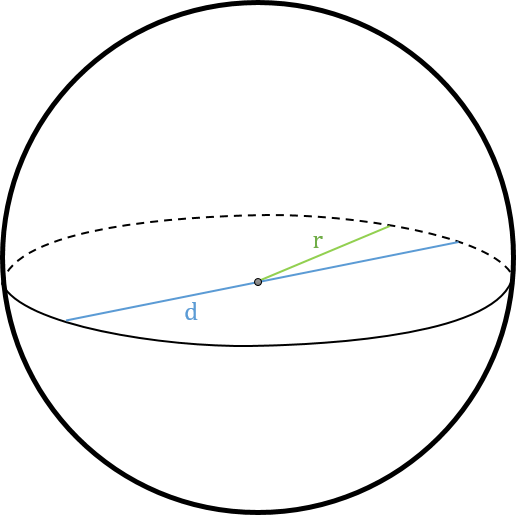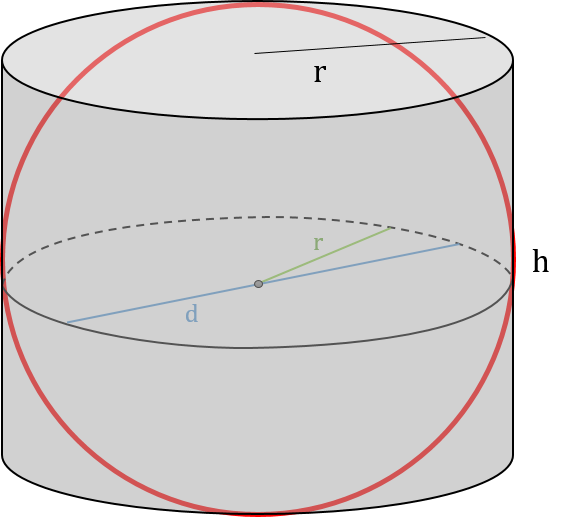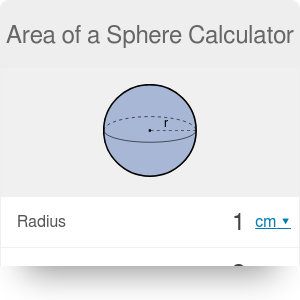in
Diameter
in
Volume
cu in
Surface to volume ratio
1/
in
Surface area
Surface area
in²

# Area of a Sphere Calculator

By Dominik Czernia, PhD candidate

With our area of a sphere calculator, you can analyze every parameter of a sphere you want, but it is chiefly dedicated to its surface area. How to find the area of a sphere? If you only want to estimate its value, enter one of the chosen quantity into the right field. However, if you want to learn about the the area of the sphere formulas, keep reading the following text.

A sphere is a three dimensional (3D) object, the contours and plane sections of which are circles. In other words, all of the points on the sphere are at the same distance from a fixed point (center). This area of a sphere calculator uses many quantities and notation is as follows:A sphere is a special object that has the lowest surface to volume ratio among all other closed surfaces with a given volume. It is just like a circle which encloses the largest area with a given perimeter compared to the other flat figures. If you want more general information about spheres, head on to the sphere calc!

We can divide any sphere into two equal parts, which are called hemispheres. Equations that describe hemisphere are very similar to those which we have presented in the below sections for a full sphere. Are you curious what are properties of the hemispheres? Check out our area and volume of a hemisphere calculators and learn more about that kind of objects!

## How to find the area of a sphere?

The first person who answered the question how to find the area of a sphere was ancient Greek - Archimedes. He had discovered that the orthogonal projection from the lateral area of a cylinder onto the sphere keeps its area. Looking in the picture below, you can see that both objects have the same radius r and the height of a cylinder equals the diameter of a sphere d. Therefore, using the lateral surface area of a cylinder formula, we obtain the following:`A = 2 * π * r * h`,

`A = 2 * π * r * d`, and since `d = 2 * r`:

`A = 2 * π * r * 2 * r`,

`A = 4 * π * r²`,

which is well-known area of a sphere formula.

## What is the area of a sphere formula?

The above-presented area of a sphere formula is written in terms of radius. However, how to find the area of a sphere when we don't have the radius `r`, but a different quantity that describes that sphere? At first, let us remind equations for those possible quantities:

1. Diameter of a sphere: `d = 2 * r`,
2. Volume of a sphere: `V = 4/3 * π * r³`,
3. Surface to volume ratio of a sphere: `A / V = 3 / r`.

Now we can try to derive various surface area of a sphere formulas. In this area of a sphere calculator we use four equations:

1. Given radius: `A = 4 * π * r²`,
2. Given diameter: `A = π * d²`,
3. Given volume: `A = ³√(36 * π * V²)`,
4. Given surface to volume ratio: `A = 36 * π / (A/V)²`.

Our area of a sphere calculator allows you to calculate the area in many different units including SI and imperial units. Also, if you want to learn how to estimate surface areas of other figures, check out our surface area calculator which is a more general tool.

Dominik Czernia, PhD candidate

## Get the widget!

Area of a Sphere Calculator can be embedded on your website to enrich the content you wrote and make it easier for your visitors to understand your message.

It is free, awesome and will keep people coming back!